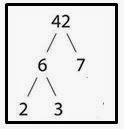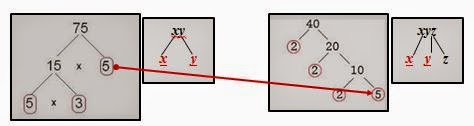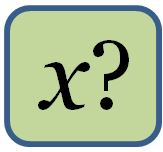### Algebraic Terms - Finding the Greatest Common Factor and Least Common Multiple Using a Venn Diagram

I tutor math at the college where I teach. Many of those students have been confused on how to find the greatest common factor for a set of algebraic terms. Having an elementary background, I introduce them to a factor tree which, believe it or not, many have never seen.

When just a rule is given by an instructor, often times, students get lost in the mathematical process. I have found that utilizing a visual can achieve an understanding of a concept better than just a rule. A Venn Diagram is such a visual and helps students to follow the process and understand the connection and relationship between each step of finding the GCF and LCM.It's important to always begin with the definitions for the
words factor, greatest common factor and least common multiple. If a student doesn't know the vocabulary, they can't do the work! I continue by explaining and illustrating what a factor tree is (on your left) and how to construct and use a Venn Diagram as a graphic organizer.Let's suppose we have the algebraic terms of 75xy and 45xyz. I have the students construct factor trees for each of the numbers as illustrated on the left.Then all the common factors are placed in the intersection of the two circles. In this case, it would be the 5 and the xy.

The students then put the remaining factors and variables in the correct big circle. Five and three would go in the left hand circle and the three 2’s and the z would be placed in the right hand circle.

The intersection is the GCF; so, the GCF for 75xy and 40xyz is 5xy.   To find the LCM, multiply the number(s) in the first big circle by the GCF (numbers in the intersection) times the number (s) in the second big circle.

5 × 3 × GCF × 2 × 2 × 2 × z = 15 × 5xy × 8z = 240. The LCM is 600xyzFree Item
This method is applicable and helpful in algebra when students are asked to find the LCM or GCF of a set of algebraic terms such as: 25xy, 40xyz. (LCM = 200xyz; GCF = 5xy) or when they must factor out the GCF from a polynomial such as 6x2y+ 9xy2. Using a Venn Diagram is also an effective and valuable tool when teaching how to reduce fractions.

Are you interested in finding out more about this method?  Then download my newest free resource entitled: Algebraic Terms and Fractions - Finding the Greatest Common Factor and the Lowest Common Multiple Using a Venn Diagram.

### Why is 'x' Usually the Unknown in Algebra?Ted Talk
Again, it's time for some math information you might have missed in school. (Don't worry, I missed a great deal as well.)  Today's question is: Why is the letter "x" the symbol usually used for an unknown?

Even though the letter "x" is commonly used in mathematics, its use often appears in non-numerical areas within different industries such as The X Files or Project X. Terry Moore clears up this mathematical mystery in a TED Talk presentation at Long Beach, California.  In a short and funny four minute talk, he gives an unexpected answer to "why." Just click under the illustration to find out the reason!